Courses

# Central Limit Theorem - Mathematical Methods of Physics, UGC - NET Physics Physics Notes | EduRev

## Physics for IIT JAM, UGC - NET, CSIR NET

Created by: Akhilesh Thakur

## Physics : Central Limit Theorem - Mathematical Methods of Physics, UGC - NET Physics Physics Notes | EduRev

The document Central Limit Theorem - Mathematical Methods of Physics, UGC - NET Physics Physics Notes | EduRev is a part of the Physics Course Physics for IIT JAM, UGC - NET, CSIR NET.
All you need of Physics at this link: Physics

We don't have the tools yet to prove the Central Limit Theorem, so we'll just go ahead and state it without proof.

Central Limit Theorem. Let X1, X2, ... , Xn be a random sample from a distribution (any distribution!) with (finite) mean μ and (finite) variance σ2. If the sample size n is "sufficiently large," then:

(1) the sample mean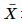follows an approximate normal distribution

(2) with mean E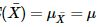(3) and variance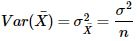We write: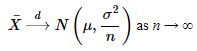or: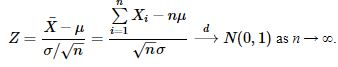So, in a nutshell, the Central Limit Theorem (CLT) tells us that the sampling distribution of the sample mean is, at least approximately, normally distributed, regardless of the distribution of the underlying random sample. In fact, the CLT applies regardless of whether the distribution of the Xi is discrete (for example, Poisson or binomial) or continuous (for example, exponential or chi-square). Our focus in this lesson will be on continuous random variables. In the next lesson, we'll apply the CLT to discrete random variables, such as the binomial and Poisson random variables.

You might be wondering why "sufficiently large" appears in quotes in the theorem. Well, that's because the necessary sample size n depends on the skewness of the distribution from which the random sample Xi comes:

1. If the distribution of the Xi is symmetric, unimodal or continuous, then a sample size n as small as 4 or 5 yields an adequate approximation.

2. If the distribution of the Xi is skewed, then a sample size n of at least 25 or 30 yields an adequate approximation.

3. If the distribution of the Xi is extremely skewed, then you may need an even larger n.

We'll spend the rest of the lesson trying to get an intuitive feel for the theorem, as well as applying the theorem so that we can calculate probabilities concerning the sample mean.

159 docs

,

,

,

,

,

,

,

,

,

,

,

,

,

,

,

,

,

,

,

,

,

,

,

,

;